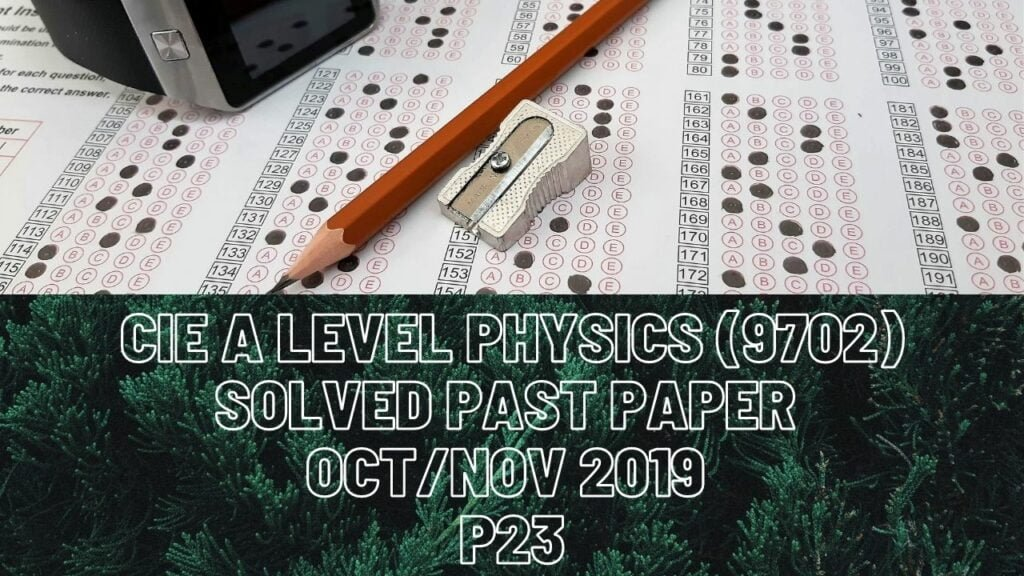A Level Physics### CIE A Level Physics Solved Past Paper Oct/Nov 2019 P23

2 bi) From t=3s to t=6s, elevator is moving with constant velocity which means there is no net force that acts on it. Thus tension in the cable that acts upwards is equal to all those forces that act downwards and they are friction and weight of the elevator. Tension=13000N of weight+2000N of friction=15000N. Work done by the motor= tension in the cable*height covered in this time interval (which can be calculated from area under graph of fig.2.2).

iii) From t=0 to t=7s, not all the energy output from motor will be converted to energy in the form of g.p.e of lift.

3 bii) Weight of the charged particle acts downwards and electric force which is due to attraction acts upwards hence resultant force is the difference between them.

4 aii) Total momentum before collision in y-direction = total momentum after collision in y-direction. Before collision, ball X has momentum of (0.3*-Vxsin(60)) in y-direction and that of ball Y is (0.2*6sin(60)) in y-direction. After collision, both the balls have zero momentum in y-direction.

6 ai) When 0.5A of current enters the loop, it splits into 0.2A and 0.3A which means that here starts the parallel connection, 28ohms of resistor and R are in series and both lamps are in series too. In series connection, current is the same for both components and total voltage gets shared. Lamps with equal resistances mean equal voltages too.